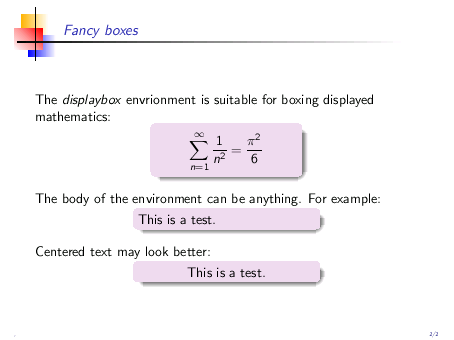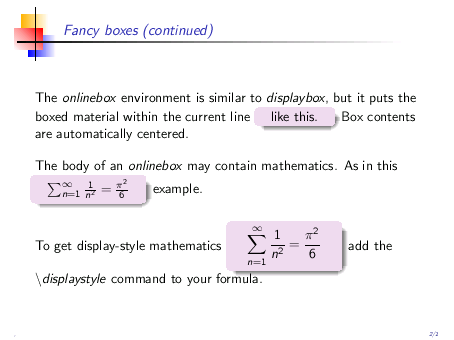# 11  UMBC boxes

The package umbcboxes helps you display text and equations in fancy boxes with rounded corners and shadows like those shown in the following samples.The package umbcboxes defines two environments: displaybox and onlinebox.

The displaybox environment is meant to be used with displayed math, however anything else can be boxed with it as well, as the examples below illustrate.

The onlinebox is meant to be used within the running text.

These environments don’t attempt to guess the widths of the desired boxes—you are expected to specify their widths, typically after some trial and error. This is not such a bad thing; finding an aesthetically pleasing box size is not a job for a computer.

You can get a flavor of the usage of these environments from these fragments.

Displayed math:

\begin{displaybox}{5cm}     % width of the box is 5cm
$\cos^2 x + \sin^2 x = 1$
\end{displaybox}


Online material:

The important formula, \begin{onlinebox}{3cm} $\cos^2 x + \sin^2 x = 1$ \end{onlinebox} occurs very frequently
in mathematics.


In specifying box widths, it is useful to have in mind that the overall size of a Beamer slide is 128mm x 96mm.

The background color of the boxes is set to structure!15!bg by default. (See The structure color and All about colors for the meaning of this.) The default color may not be particularly exciting in general, therefore it is likely that you will want to change it to something more interesting. The \setbeamercolor command in the illustration below shows how.

Here is the complete code that produced the two slides shown at the top of this page.

% umbcboxes-demos.tex
\documentclass{beamer}
\usetheme{umbc4}
\useinnertheme{umbcboxes}
\setbeamercolor{umbcboxes}{bg=violet!15,fg=black}  % redefine box color!
\begin{document}

\begin{frame}{Fancy boxes}

The \textsl{displaybox} environment is suitable for boxing
displayed mathematics:
\begin{displaybox}{4cm}
$\sum_{n=1}^\infty \frac{1}{n^2} = \frac{\pi^2}{6}$
\end{displaybox}
\bigskip

The body of the environment can be anything.  For example:
\begin{displaybox}{5cm}
This is a test.
\end{displaybox}
\bigskip

Centered text may look better:
\begin{displaybox}{5cm}
\centerline{This is a test.}
\end{displaybox}

\end{frame}

\begin{frame}{Fancy boxes (continued)}

The \textsl{onlinebox} environment is similar to \textsl{displaybox},
but it puts the boxed material within the current line
\begin{onlinebox}{2cm} like this.\end{onlinebox}
Box contents are automatically centered.
\bigskip

The body of an \textsl{onlinebox} may contain mathematics.  As in this
\begin{onlinebox}{3cm}
$\sum_{n=1}^\infty \frac{1}{n^2} = \frac{\pi^2}{6}$
\end{onlinebox} example.
\bigskip

To get display-style mathematics
\begin{onlinebox}{3cm}
$\displaystyle \sum_{n=1}^\infty \frac{1}{n^2} = \frac{\pi^2}{6}$
\end{onlinebox}
add the \textsl{$\backslash$displaystyle} command to your formula.

\end{frame}

\end{document}